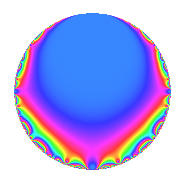# Properties

 Label 31.1.bLevel 31 Weight 1 Character orbit b Rep. character $$\chi_{31}(30,\cdot)$$ Character field $$\Q$$ Dimension 1 Newforms 1 Sturm bound 2 Trace bound 0

# Related objects

## Defining parameters

 Level: $$N$$ = $$31$$ Weight: $$k$$ = $$1$$ Character orbit: $$[\chi]$$ = 31.b (of order $$2$$ and degree $$1$$) Character conductor: $$\operatorname{cond}(\chi)$$ = $$31$$ Character field: $$\Q$$ Newforms: $$1$$ Sturm bound: $$2$$ Trace bound: $$0$$

## Dimensions

The following table gives the dimensions of various subspaces of $$M_{1}(31, [\chi])$$.

Total New Old
Modular forms 2 2 0
Cusp forms 1 1 0
Eisenstein series 1 1 0

The following table gives the dimensions of subspaces with specified projective image type.

$$D_n$$ $$A_4$$ $$S_4$$ $$A_5$$
Dimension 1 0 0 0

## Trace form

 $$q$$ $$\mathstrut -\mathstrut q^{2}$$ $$\mathstrut -\mathstrut q^{5}$$ $$\mathstrut -\mathstrut q^{7}$$ $$\mathstrut +\mathstrut q^{8}$$ $$\mathstrut +\mathstrut q^{9}$$ $$\mathstrut +\mathstrut O(q^{10})$$ $$q$$ $$\mathstrut -\mathstrut q^{2}$$ $$\mathstrut -\mathstrut q^{5}$$ $$\mathstrut -\mathstrut q^{7}$$ $$\mathstrut +\mathstrut q^{8}$$ $$\mathstrut +\mathstrut q^{9}$$ $$\mathstrut +\mathstrut q^{10}$$ $$\mathstrut +\mathstrut q^{14}$$ $$\mathstrut -\mathstrut q^{16}$$ $$\mathstrut -\mathstrut q^{18}$$ $$\mathstrut -\mathstrut q^{19}$$ $$\mathstrut +\mathstrut q^{31}$$ $$\mathstrut +\mathstrut q^{35}$$ $$\mathstrut +\mathstrut q^{38}$$ $$\mathstrut -\mathstrut q^{40}$$ $$\mathstrut -\mathstrut q^{41}$$ $$\mathstrut -\mathstrut q^{45}$$ $$\mathstrut +\mathstrut 2q^{47}$$ $$\mathstrut -\mathstrut q^{56}$$ $$\mathstrut -\mathstrut q^{59}$$ $$\mathstrut -\mathstrut q^{62}$$ $$\mathstrut -\mathstrut q^{63}$$ $$\mathstrut +\mathstrut q^{64}$$ $$\mathstrut +\mathstrut 2q^{67}$$ $$\mathstrut -\mathstrut q^{70}$$ $$\mathstrut -\mathstrut q^{71}$$ $$\mathstrut +\mathstrut q^{72}$$ $$\mathstrut +\mathstrut q^{80}$$ $$\mathstrut +\mathstrut q^{81}$$ $$\mathstrut +\mathstrut q^{82}$$ $$\mathstrut +\mathstrut q^{90}$$ $$\mathstrut -\mathstrut 2q^{94}$$ $$\mathstrut +\mathstrut q^{95}$$ $$\mathstrut -\mathstrut q^{97}$$ $$\mathstrut +\mathstrut O(q^{100})$$

## Decomposition of $$S_{1}^{\mathrm{new}}(31, [\chi])$$ into irreducible Hecke orbits

Label Dim. $$A$$ Field Image CM RM Traces $q$-expansion
$$a_2$$ $$a_3$$ $$a_5$$ $$a_7$$
31.1.b.a $$1$$ $$0.015$$ $$\Q$$ $$D_{3}$$ $$\Q(\sqrt{-31})$$ None $$-1$$ $$0$$ $$-1$$ $$-1$$ $$q-q^{2}-q^{5}-q^{7}+q^{8}+q^{9}+q^{10}+\cdots$$